International
Tables for
Crystallography
Volume B
Reciprocal space
Edited by U. Shmueli

International Tables for Crystallography (2010). Vol. B, ch. 1.5, pp. 182-184   | 1 | 2 |

## Section 1.5.5.1. Guide to the figures

M. I. Aroyoa* and H. Wondratschekb

aDepartamento de Fisíca de la Materia Condensada, Facultad de Cienca y Technología, Universidad del País Vasco, Apartado 644, 48080 Bilbao, Spain , and bInstitut für Kristallographie, Universität, D-76128 Karlsruhe, Germany
Correspondence e-mail:  wmpararm@lg.ehu.es

#### 1.5.5.1. Guide to the figures

| top | pdf |

Each figure caption gives the name of the arithmetic crystal class of space groups to which the Brillouin zone belongs. If there is more than one figure for this arithmetic crystal class, then these figures refer to different geometric conditions for the lattice. Therefore, for each of the figures the arithmetic crystal class is followed by the specific conditions for the lattice parameters of this figure, e.g.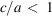' for Fig. 1.5.5.3or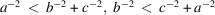and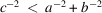' for Fig. 1.5.5.5.

Then the space groups of the arithmetic crystal class are listed with their Hermann–Mauguin symbols, their Schoenflies symbols and their space-group numbers in IT A in parentheses. Following this the type of the reciprocal-space group is denoted, e.g., No. 44' for the arithmetic crystal class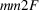in Fig. 1.5.5.5, together with the conditions for the lattice parameters of the reciprocal lattice, if any, and the number of the corresponding table.

The Brillouin zones are objects in reciprocal space. They are displayed in the figures. The reciprocal space is a vector space and its elements are the k vectors. Thus the Brillouin zone is a construction in vector space. Because the Brillouin zones are visualized by drawings consisting of vertices, lines and planes, one usually speaks of points, lines and planes in or on the border of the Brillouin zone, not of vectors. Here we follow this tradition.

The Brillouin zones are projected onto the drawing plane by a clinographic projection which may be found e.g. in Smith (1982), pp. 61 f. The coordinate axes are designated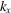,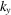and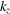; the-coordinate axis points upwards in the projection plane. The diagrams of the Brillouin zones follow those of CDML in order to facilitate the comparison of the data. The origin O with coordinates 0, 0, 0 always forms the centre of the Brillouin zone and is called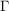.

A minimal domain is the smallest fraction of the Brillouin zone which contains exactly one wavevector k from each orbit. In these examples, the representation domain of CDML is compared with the minimal domain, called asymmetric unit', of the Bilbao Crystallographic Server. This asymmetric unit is a simple body and is often chosen in analogy to that of IT A. It may coincide with the representation domain of Table 3.10 in CDML, but is mostly rather different. Other than the representation domains of CDML, the asymmetric unit is often not fully contained in the Brillouin zone but protrudes from it, in particular by flagpoles and wings, cf. the end of this section.

In the figures the edges of the chosen asymmetric unit are drawn into the frame of the Brillouin zone. The names of points, lines and planes of CDML are retained in this listing. New names have been given to points and lines which are not listed in CDML.

The shape of the Brillouin zone depends on the lattice relations. Therefore, there may be vertices of the Brillouin zone with a variable coordinate. If such a point is displayed and designated in a figure by an upper-case letter, then the label of its variable coordinate in the corresponding table is the same letter but lower case. Thus, the variable coordinate of the point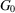is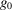, of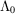is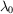etc.

In CDML, the same letter may designate items of different quality in different figures and tables. For example, there is a point H in Fig. 1.5.5.1and Table 1.5.5.1but a line H in Fig. 1.5.5.5and Table 1.5.5.5. In the figures and tables of these examples not only lines and points but also their equivalent objects are listed and the parameter ranges of the lines are described. Therefore, the endpoints of the line H, the points equivalent to a point H as well as the lines equivalent to a line H may be also designated by the letter H but distinguished by indices. In order to recognize points and lines easily, the indices of points are always even: H0, H2, H4; those of lines are always odd: H1, H3.

A point is marked in a figure by its name and by a black circle filled with white if it is listed in the corresponding k-vector table but is not a point of special symmetry. The same designation is used for the auxiliary points that have been added in order to facilitate the comparison between the two descriptions of the k-vector types. Non-coloured parts of the coordinate axes, of the edges of the Brillouin zone or auxiliary lines are displayed by thin solid black lines. Such lines are dashed or omitted if they are not visible, i.e. are hidden by the body of the Brillouin zone or of the asymmetric unit.

The representatives for the orbits of symmetry points or of symmetry lines, as well as the edges of the representation domain of CDML and of the chosen asymmetric unit are shown in colour.

• (a) A representative point of each orbit of symmetry points is designated by a red- or cyan-filled circle with its name also in red or cyan if it belongs to the asymmetric unit or to the representation domain of CDML. If both colours could be used, e.g. if the asymmetric unit coincides with the representation domain, the colour is red.

Note that a point is coloured red or cyan only if it is really a symmetry point, i.e. its little co-group is a proper supergroup of the little co-groups of all points in its neighbourhood. Such a point has no variable parameters in its coordinates. Points listed by CDML are not coloured if they are part of a symmetry line or symmetry plane only.

• (b) Coloured lines are always broad. They are solid lines if they are `visible', i.e. if they are not hidden by the Brillouin zone or by the asymmetric unit. A hidden symmetry line or edge of the asymmetric unit is not suppressed but is coloured as a dashed line.

• (c) The meanings of the different coloured lines and the names used for them in the text are as follows:Notes:

 (1) The colour of the line is pink for an edge of the asymmetric unit which is not a symmetry line. (2) The colour is red for a symmetry line of the asymmetric unit, with the name also in red. (3) The colour of the line is brown with the name in red for a line which is a symmetry line as well as an edge of the asymmetric unit.

The representation domain of CDML is displayed in the same figure.

 (1) The edges of the representation domain are coloured light blue. (2) The symmetry points and lines with their letters are coloured cyan. (3) Edges of the representation domain or common edges of the representation domain and the asymmetric unit are coloured dark blue with the letters in cyan if they are symmetry lines of the representation domain but not of the asymmetric unit. Common edges of an asymmetric unit and a representation domain are coloured pink if they are not symmetry lines simultaneously.

Exactly one element of each point orbit, line orbit or orbit of planes is contained in the asymmetric unit. Exceptionally, different elements of the same orbit have been coloured because of their special meaning. In these cases the different elements are connected in the corresponding table by the equivalence sign, see, e.g. the lines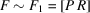or the planes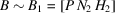in Table 1.5.5.1.

To enable a uni-arm description, symmetry lines outside the asymmetric unit may be selected as orbit representatives. Such a piece of a line is called a flagpole. Flagpoles are always coloured red, see, e.g., the line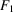in Fig. 1.5.5.1.

Symmetry planes are not distinguished in the figures. However, in analogy to the flagpoles, symmetry planes outside the asymmetric unit may be selected as orbit representatives. Such a piece of a plane is called a wing. Wings are always coloured pink, see, e.g., Fig. 1.5.5.1.

Within the caption of each figure the following data are listed:

 (i) a statement of whether the representation domain and the asymmetric unit are identical or not; (ii) the coordinates of auxiliary points if not specified in the corresponding table; (iii) the parameter descriptions of the flagpoles and the wings.

### References

Smith, J. V. (1982). Geometrical and Structural Crystallography. New York: John Wiley & Sons.